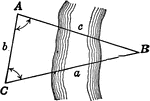### Oblique Triangle for Distance Across a River

Illustration of oblique triangle used to find distance across a river.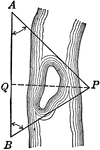### Oblique Triangle for Distance Across a River

Illustration of oblique triangle used to find distance across a river.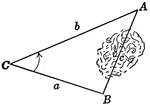### Oblique Triangle for Distance Across Lake

Illustration of oblique triangle used to find distance across a lake.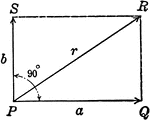### Resultant Vector With Vectors at 90 degrees

Illustration of the resultant vector when two vectors are acting upon a body at point P at 90 degrees.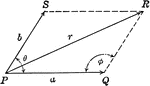### Resultant Vector With Vectors at Angle Ǝ

Illustration of the resultant vector when two vectors are acting upon a body at point P at Ǝ degrees.